• 误差反向传播
2021-08-22 20:43:05

### 原理

误差反向传播算法是通过误差函数计算实际输出值与期望输出值之间的误差，把误差从最后的输出层依次传播到之前各层，最后通过调整各层连接权重与偏置达到减小误差的目的。而权重和偏置的调整一般使用梯度下降法。

### 推导前的准备

本文误差反向传播算法的推导以包含隐藏层的多层感知器为例，结构如下图所示：这里，图中和推导过程中涉及的符号代表的含义分别为：

符号含义
x i x_i 输入值
a j a_j 隐藏层激活值
y k y_k 实际输出值
r k r_k 期望输出值
w 1 i j w_{1ij} 网络输入层的第i个神经元和下一层网络第j个神经元之间的连接权重
w 2 j k w_{2jk} 网络隐藏层的第j个神经元和下一层网络第k个神经元之间的连接权重
b j 2 b_j^2 网络隐藏层第j个神经元的偏置
b k 3 b_k^3 网络输出层第k个神经元的偏置
u 1 j u_{1j} 隐藏层第j个神经元的激活函数的加权输入
u 2 k u_{2k} 输出层第k个神经元的激活函数的加权输入
E E 误差函数
η \eta 学习率

这里，误差函数采用最小二乘误差函数，公式如下：
E = ∑ n = 1 N ∣ ∣ r n − y n ∣ ∣ 2 E=\sum_{n=1}^N||r_n-y_n||^2
激活函数使用sigmoid函数，公式如下：
f ( u ) = 1 1 + e − u f(u)=\frac{1}{1+e^{-u}} 其中，
u = ∑ i = 1 N w i x i + b u=\sum_{i=1}^Nw_ix_i+b

### 开始推导

首先，再明确一下我们的目的是计算使用梯度下降法时神经网络各层间连接权重和偏差的变化值，即 Δ w 1 i j , Δ w 2 j k , Δ b j 2 \Delta w_{1ij},\Delta w_{2jk},\Delta b_j^2 等值。
这里，计算过程以求解 Δ w 1 i j \Delta w_{1ij} 为例，其它值类似，而
Δ w 1 i j = η ∂ E ∂ w 1 i j \Delta w_{1ij}=\eta\frac{\partial E}{\partial w_{1ij}}
根据链式求导得：
∂ E ∂ w 1 i j = ∑ k = 1 q ( ∂ E ∂ y k ∂ y k ∂ u 2 k ∂ u 2 k ∂ a j ∂ a j δ u 1 j ∂ u 1 j ∂ w 1 i j ) \frac{\partial E}{\partial w_{1ij}}=\sum_{k=1}^q (\frac{\partial E}{\partial y_k} \frac{\partial y_k}{\partial u_{2k}} \frac{\partial u_{2k}}{\partial a_j} \frac{\partial a_j}{\delta u_{1j}} \frac{\partial u_{1j}}{\partial w_{1ij}})
然后逐个对等式右侧各部分求解得：
∂ E ∂ y k = − ( r k − y k ) \frac{\partial E}{\partial y_k}=-(r_k-y_k)
∂ y k ∂ u 2 k = f ( u 2 k ) ( 1 − f ( u 2 k ) ) = y k ( 1 − y k ) \frac{\partial y_k}{\partial u_{2k}}=f(u_{2k})(1-f(u_{2k}))=y_k(1-y_k)
∂ u 2 k ∂ a j = w 2 j k \frac{\partial u_{2k}}{\partial a_j}=w_{2jk}
∂ a j ∂ u 1 j = a j ( 1 − a j ) \frac{\partial a_j}{\partial u_{1j}}=a_j(1-a_j)
∂ u 1 j ∂ w 1 i j = x i \frac{\partial u_{1j}}{\partial w_{1ij}}=x_i
最后得：
∂ E ∂ w 1 i j = − ∑ k = 1 q [ ( r k − y k ) y k ( 1 − y k ) w 2 j k a j ( 1 − a j ) x i ] \frac{\partial E}{\partial w_{1ij}}=-\sum_{k=1}^q [(r_k-y_k) y_k(1-y_k) w_{2jk} a_j(1-a_j) x_i]
因此，权重 w 1 i j w_{1ij} 得变化值为：
Δ w 1 i j = η ∑ k = 1 q [ ( r k − y k ) y k ( 1 − y k ) w 2 j k a j ( 1 − a j ) x i ] \Delta w_{1ij}=\eta \sum_{k=1}^q [(r_k-y_k) y_k(1-y_k) w_{2jk} a_j(1-a_j) x_i]
根据上述计算过程可以发现，权重的变化值的组成依次为：学习率、误差函数导数、激活函数导数、对应连接权重、激活函数导数和输入值，根据这个规律可以快速写出各层权重和偏差的变化值，例如：
Δ w 2 j k = η ∑ k = 1 q [ ( r k − y k ) y k ( 1 − y k ) a j ] \Delta w_{2jk} = \eta \sum_{k=1}^q [(r_k-y_k)y_k(1-y_k)aj]
Δ b j 2 = η ∑ k = 1 q [ ( r k − y k ) y k ( 1 − y k ) w 2 j k a j ( 1 − a j ) ] \Delta b_j^2 = \eta \sum_{k=1}^q[(r_k-y_k)y_k(1-y_k)w_{2jk}a_j(1-a_j)]

### 小结

误差反向传播算法会通过误差函数计算梯度，然后调整权重与偏置，经过反复迭代训练的调整，最终获得最优解。
本文在推导过程中使用的误差函数为最小二乘误差函数，除此以外还有很多其它函数用于计算误差，比如一般多分类问题中使用交叉熵误差函数：
E = − ∑ c = 1 C ∑ n = 1 N r c n l n y c n E=-\sum_{c=1}^C \sum_{n=1}^N r_{cn}lny_{cn}
二分类中的常用的误差函数为：
E = − ∑ n = 1 N [ r n l n y n + ( 1 − r n ) l n ( 1 − y n ) ] E=-\sum_{n=1}^N [r_nlny_n+(1-r_n)ln(1-y_n)]
同时，激活函数除sigmoid外还有tanh、ReLU等函数：
t a n h ( u ) = e x p ( u ) − e x p ( − u ) e x p ( u ) + e x p ( − u ) tanh(u)=\frac{exp(u)-exp(-u)}{exp(u)+exp(-u)}
R e L U ( u ) = m a x ( u , 0 ) ReLU(u)=max(u,0)深度学习 神经网络 算法 梯度下降法
更多相关内容
• 误差反向传播法构建两层人工神经网络实现mnist数据集识别，包括理解计算图的两个小例子。py文件代码
• 第四步：误差反向传播 使用误差反向传播算法对误差损失函数的梯度信息进行反向传播，同时更新所有的模型参数，首先更新输出层模型参数。 梯度下降法的参数更新公式如下： 这里要计算误差损失函数对 wo 和 bo 的偏导...

通过单个感知机或者单层神经网络只能够实现线性分类的问题，而多层神经网络可以解决非线性分类问题。

神经网络中的模型参数，是神经元模型中的连接权重以及每个功能神经元的阈值，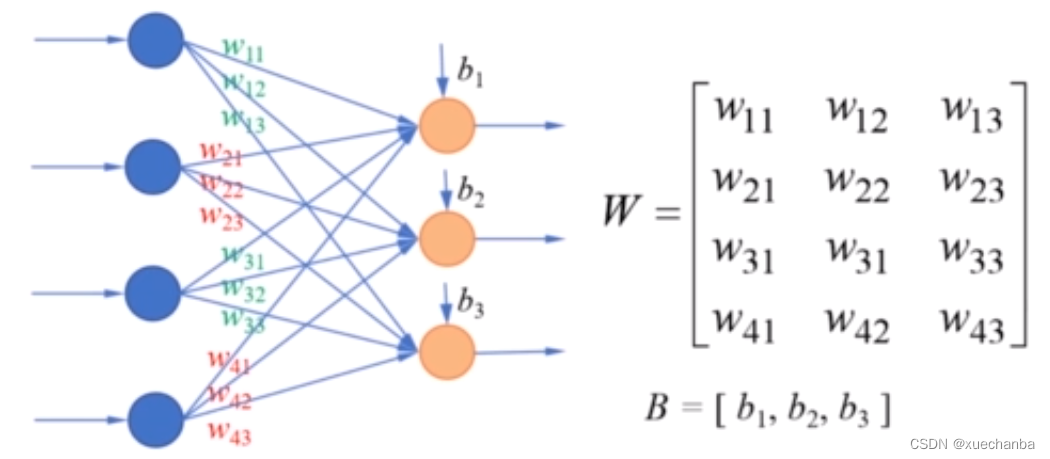这些模型参数并不是我们人工设计或者指定的，而是通过算法自动学习到的。

和其他机器学习算法一样，神经网络的训练，就是给定训练集，通过学习算法，确定学习参数的过程。可以直接使用梯度下降法需要计算损失函数的梯度，在没有隐含层的单层神经网络中，可以通过计算直接得到梯度。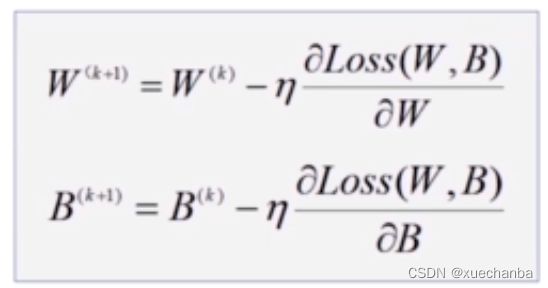在多层神经网络中，上一层的输出是下一层的输入，要在网络中的每一层计算损失函数的梯度会非常的复杂，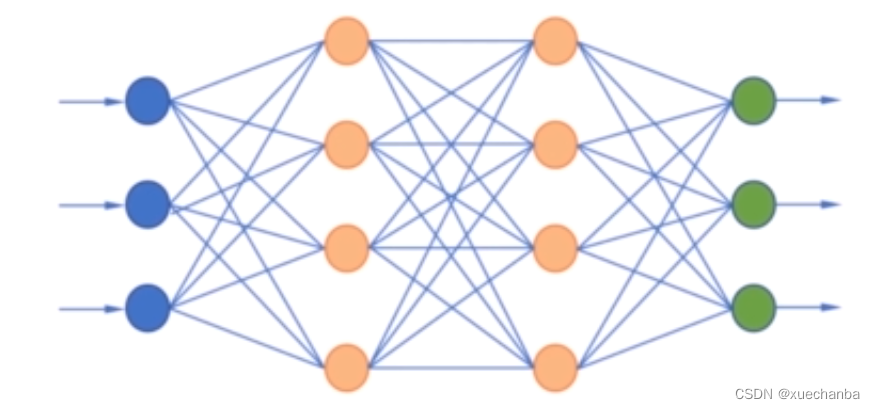为了解决这个问题，科学家提出一种解决方法，即利用链式法则，反向传播损失函数的梯度信息，只要从后往前遍历一遍神经网络，就能够计算出损失函数对网络中所有模型参数的梯度。这种方法被称之为误差反向传播算法。

需要注意的是，很多人认为误差反向传播算法是多层神经网络的学习算法。这是不对的。

误差反向传播算法仅仅是一种在神经网络的训练过程中用来计算梯度的方法，它能够计算损失函数对网络中所有模型参数的梯度，这个梯度会反馈给某种学习算法，例如梯度下降法，用来更新权值，最小化损失函数，这里梯度下降法才是学习算法，除了梯度下降法，也可以采用其他的学习算法。另外，误差反向传播法并不仅仅适应于多层神经网络，原则上它可以计算任何函数的导数。

为了便于理解，我们首先来看一个简单的例子，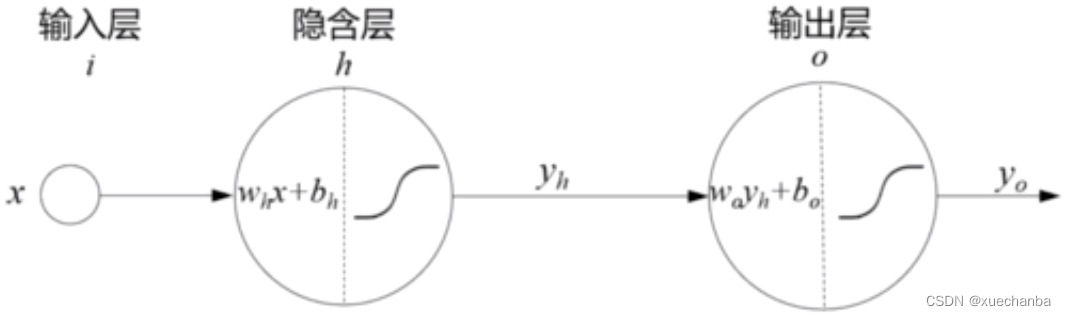上图为一个最简单的两层神经网络。它的输入层、隐含层和输出层都只有一个节点。隐含层和输出层的激活函数都使用 Sigmoid 函数，隐含层神经元接收输入值 x ，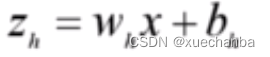首先，进行线性变换，然后将线性变换的结果 zh 作为激活函数函数的输入，从而产生隐含层的输出 yh ,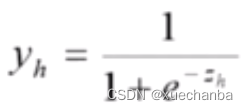输出层神经元接收隐含层的输出yh 作为输入，对其进行线性变换，产生zo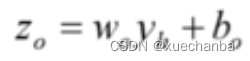然后再将 zo 作为激活函数函数的输入，从而产生神经网络的输出 yo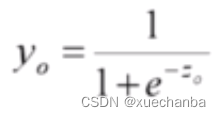这个神经网络中的所有模型参数是 wh ，bh ，wo ，bo .

训练的过程就是将样本数据ｘ输入网络中，从而通过学习算法，寻找合适的模型参数，使得网络的输出yo　与样本数据的标签一致。

假设现在有一个样本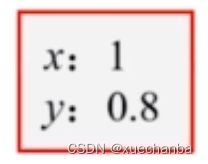我们将其输入到这个神经网络中，看看网络是如何进行训练的，
步骤一：设置模型参数初始值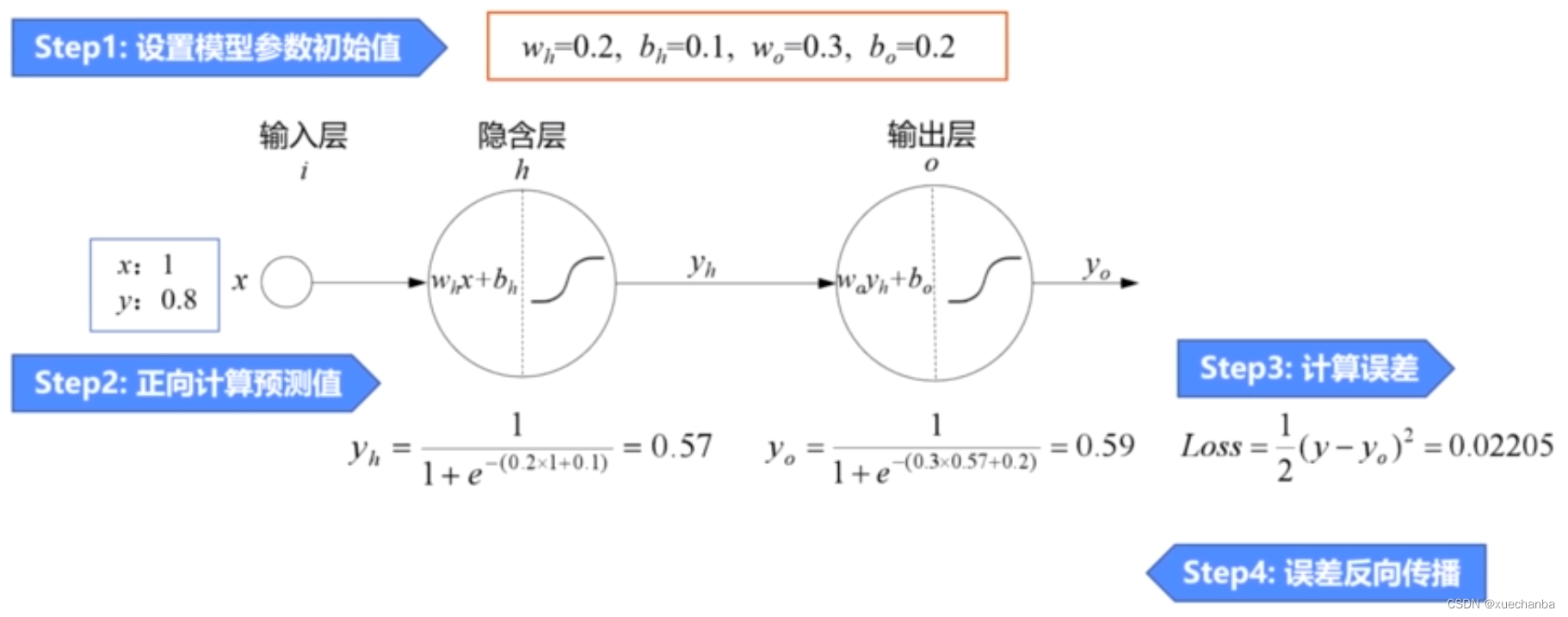步骤二：正向计算预测值
使用模型参数初始值和输入值ｘ，逐层计算，得到神经网络的预测值０.５９，它而样本标签０.８相差比较大，因此需要使用误差函数来调整模型参数，也就是训练网络。

第三步：计算误差
使用平方损失函数计算出预测值和标签值之间的误差。

第四步：误差反向传播
使用误差反向传播算法对误差损失函数的梯度信息进行反向传播，同时更新所有的模型参数，首先更新输出层模型参数。

梯度下降法的参数更新公式如下：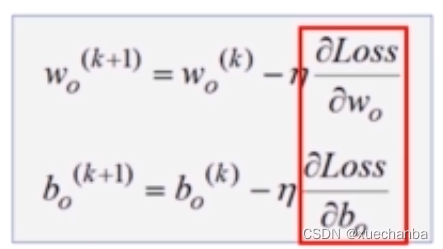这里要计算误差损失函数对 wo 和 bo 的偏导数，在之前，我们在编程实现逻辑回归时，直接使用了 TensorFlow 中提供的自动求导函数，并没有关心这个倒数是如何计算出来的。

现在，来手动计算一下，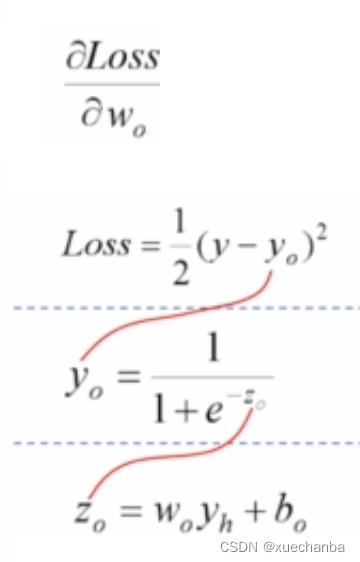可以发现，这三个函数之间是嵌套的关系，要得到损失函数对wo 的偏导数，可以使用链式求导法则来进行计算。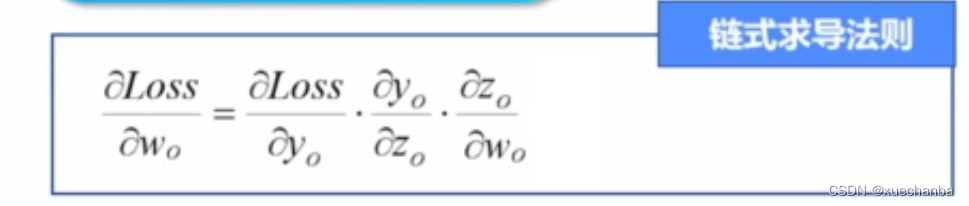下面来分别对每一项进行计算。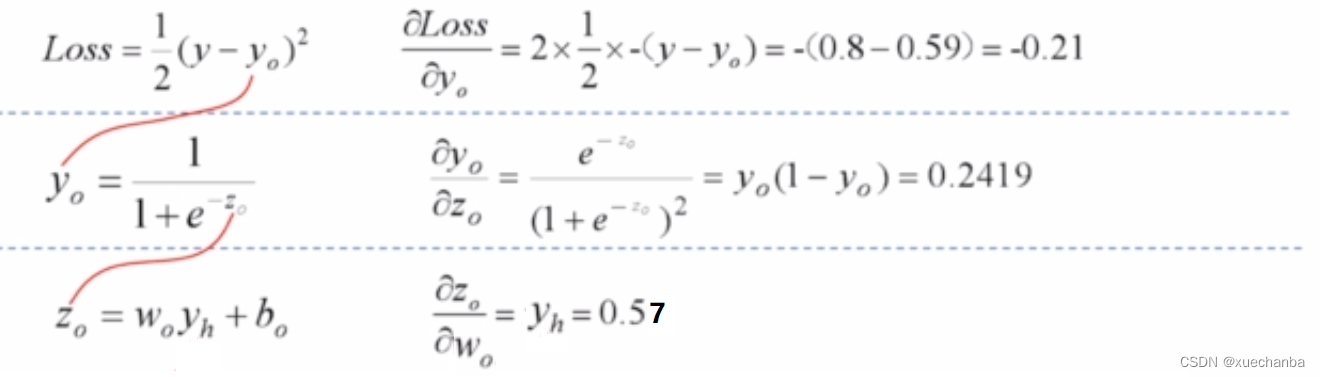再将这三个得到的值进行相乘，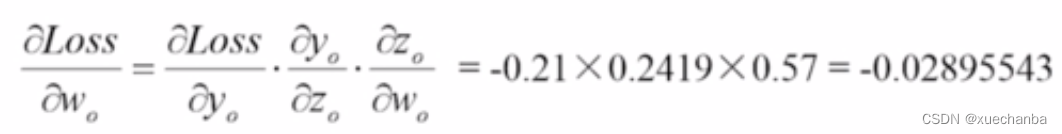就得到了损失函数对 wo 的偏导数。

取学习率 η 等于 0.5 ，根据迭代公式，更新 wo 的值。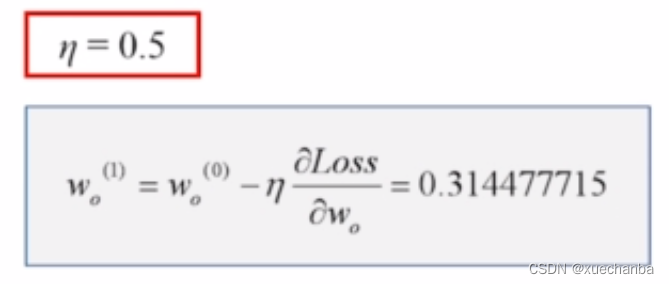同样的方法可得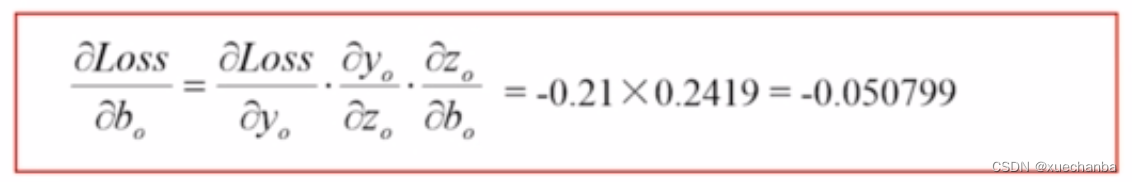其中，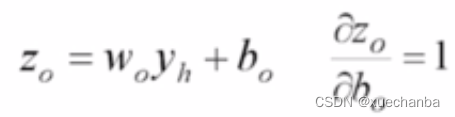再使用迭代公式更新 bo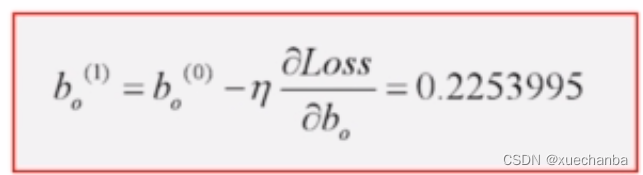现在，输出层的参数就已经更新好了。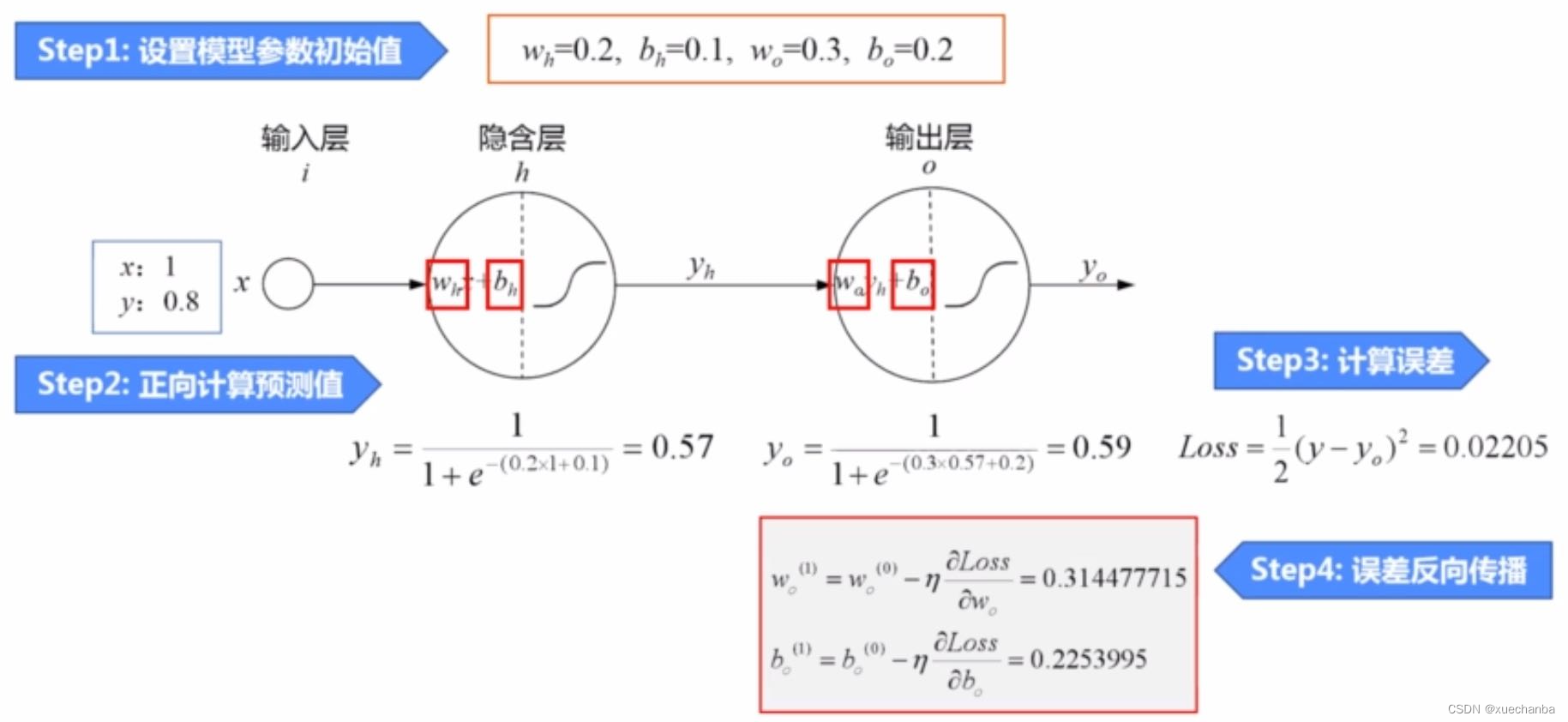下面，继续更新隐含层的参数，同样，梯度下降法的参数更新公式如下：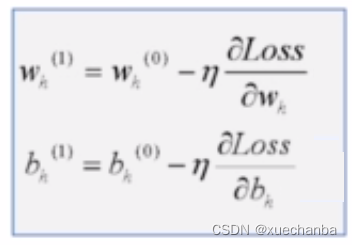这里要计算误差损失函数对 wh 和 bh 的偏导数。

同样可以使用链式求导法则来进行计算。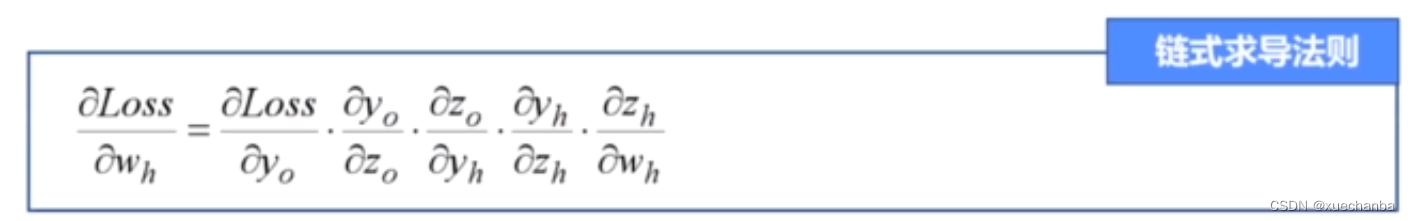并且存在下述嵌套关系，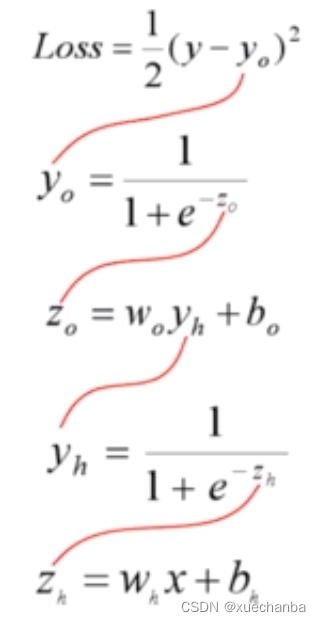计算其中所有的偏导数，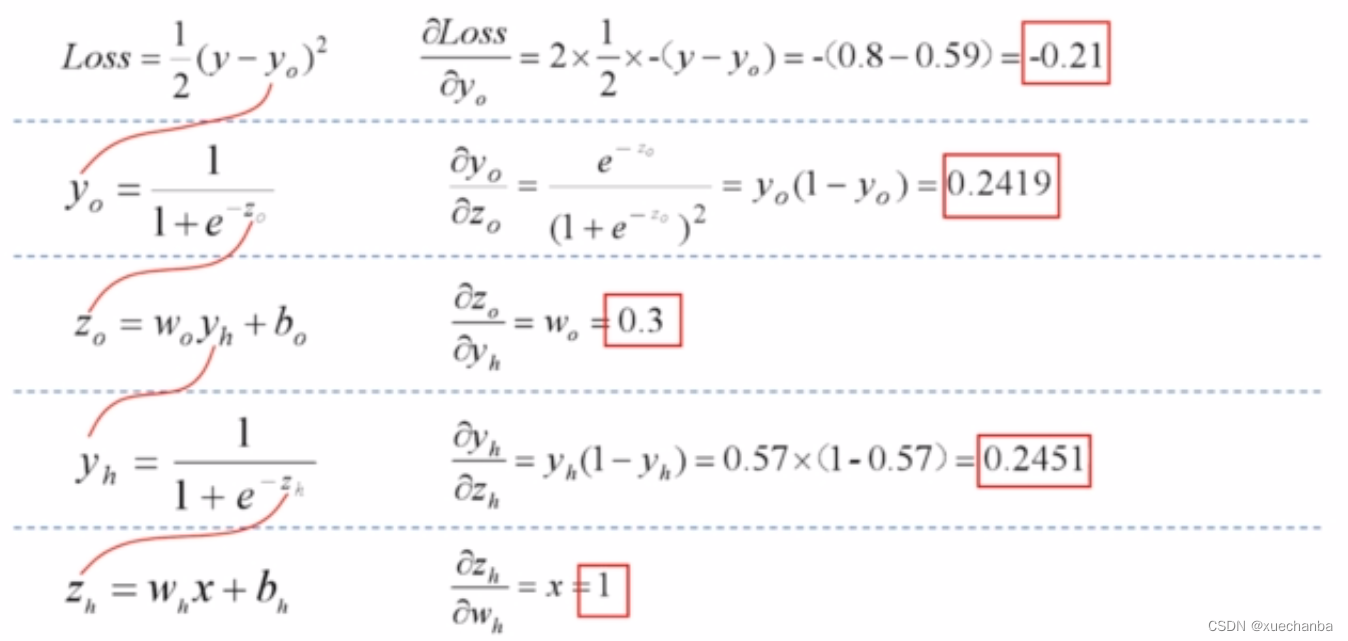最后把他们的各自结果代入上面的链式求导公式，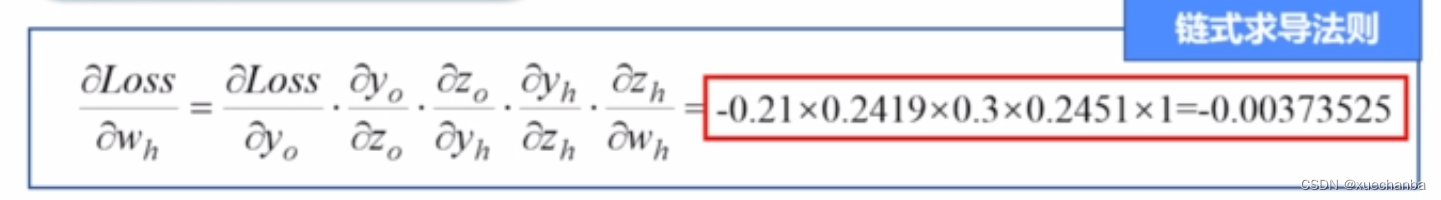最终求得损失函数对 wh 的偏导数结果，采用同样的方法，最终也可以得到损失函数对 bh 的偏导数。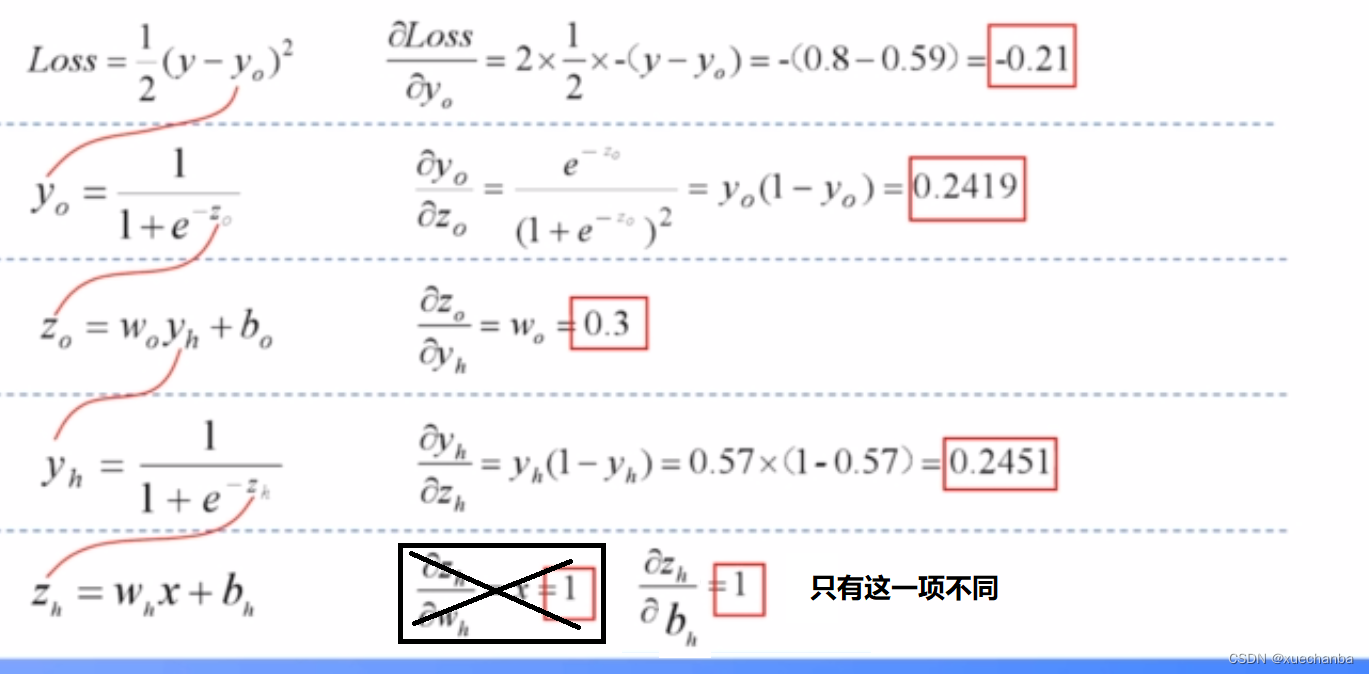(计算技巧：与损失函数对 wh 的偏导数公式相比，只有最后一项不同。)

最后代入上述公式可以得到。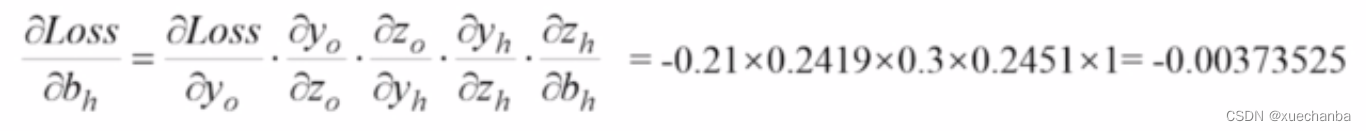得到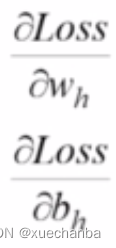之后，就可以根据迭代公式得到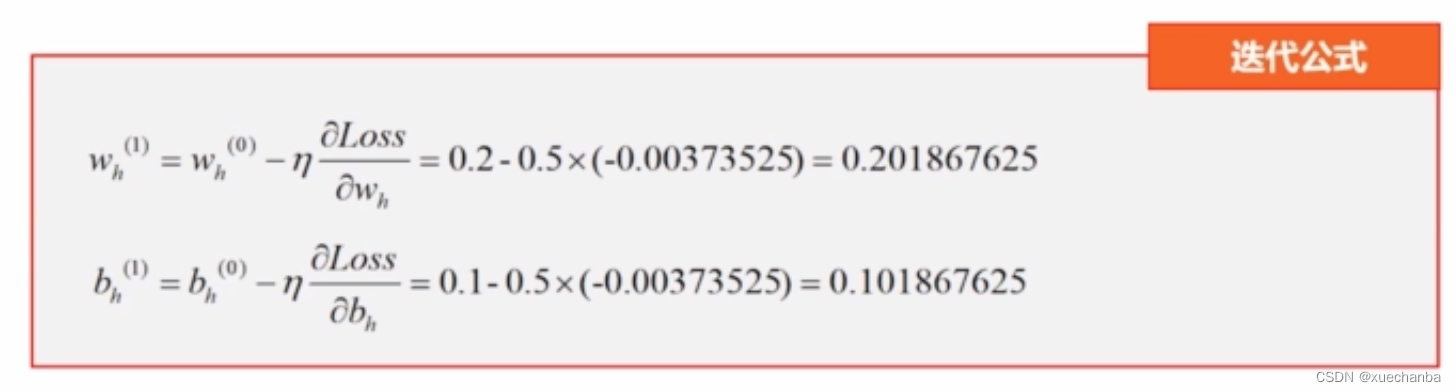到此为止，我们已经将这个神经网络中的四个模型参数都更新了，完成了一轮训练。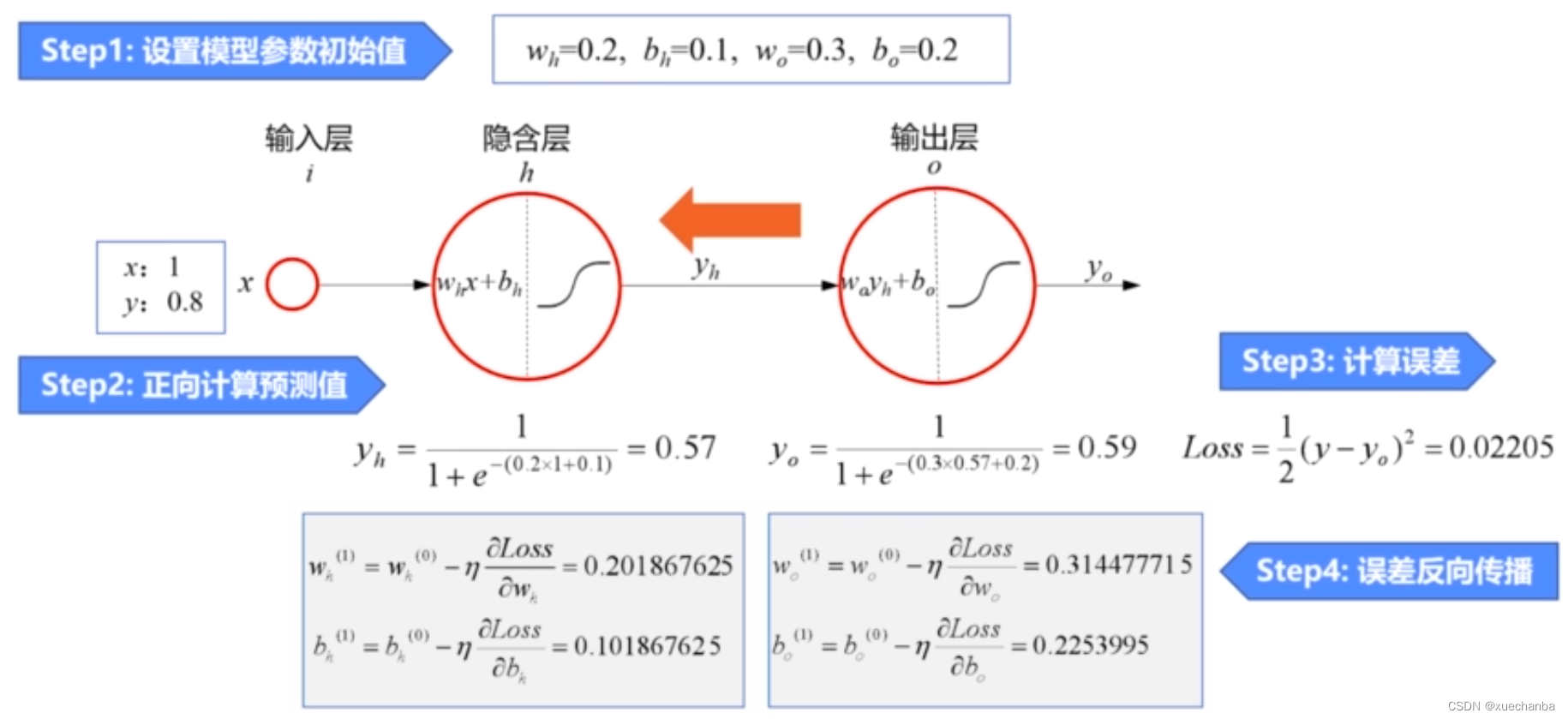接下来，再使用新的参数逐层正向计算得到新的预测值，然后和标签值进行比较计算误差，再逐层反向传播损失函数的梯度信息，更新模型参数，完成下一轮训练，如此循环，直到误差收敛到一个理想的值。

这个神经网络相对简单，每层只有一个神经元，因此，输出层的误差和梯度全部被反向传播给隐含层。

如果隐含层中有多个神经元，那么误差项就会根据不同神经元的贡献程度，进行反向传播。这个贡献程度，是由它们的权值来决定的。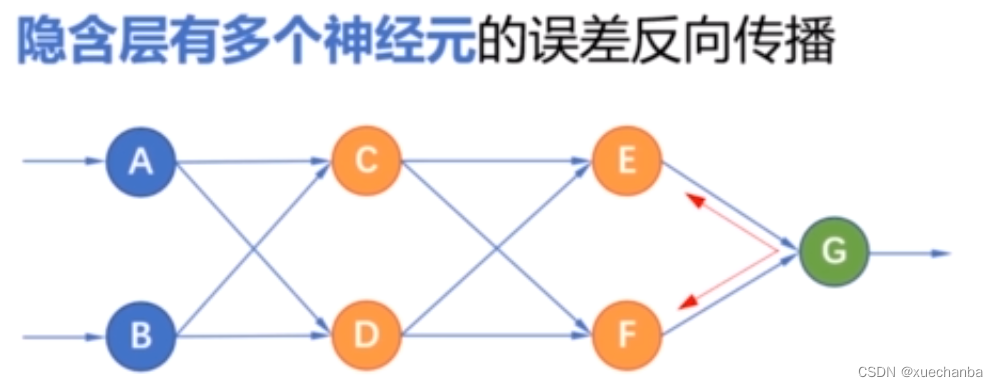例如，一个是 0.4 ，一个是 0.6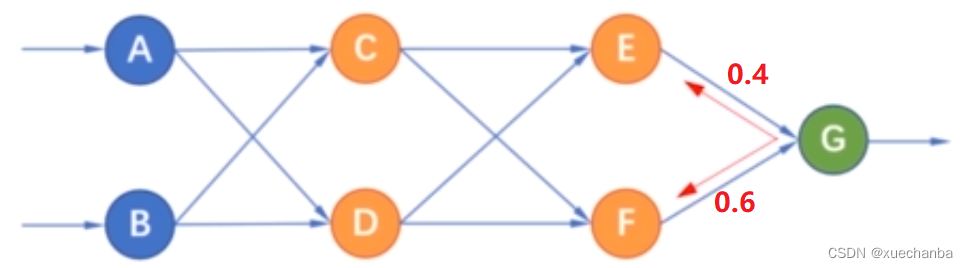那么就会把输出层的误差按照这个权值的比例分别传递给节点 E 和结点 F，同样隐含层节点 E 的误差，也按照连接的权值分别传递给节点 C 和结点 D。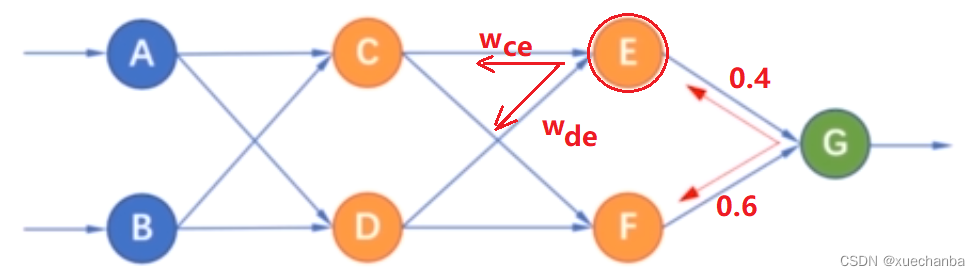下图分别是节点 C 和结点 D 接收到的来自节点 E 的误差，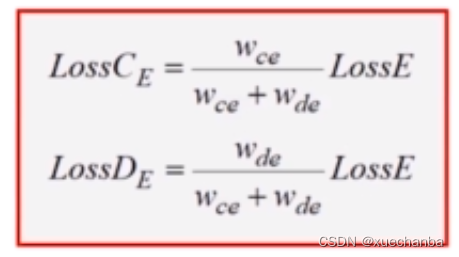结点 F 的误差也按照权值分别传递给节点 C 和结点 D，下图即为节点 C 和结点 D 接收到的来自结点 F 的误差，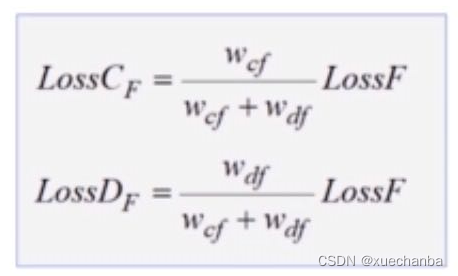对于节点 C 来说，它分别接收来自节点 E 和 F 传递过来的误差，下图为它接收到的误差，同样对于节点 D 来说，它也是分别接收来自节点 E 和 F 传递过来的误差，下图为它接收到的误差。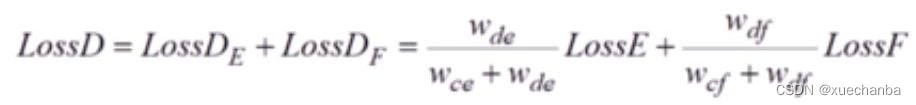当隐含层中，有多个结点时，神经网络就是这样逐层按照权值反向传播误差的。

如果再复杂一点，输出层也有两个神经元，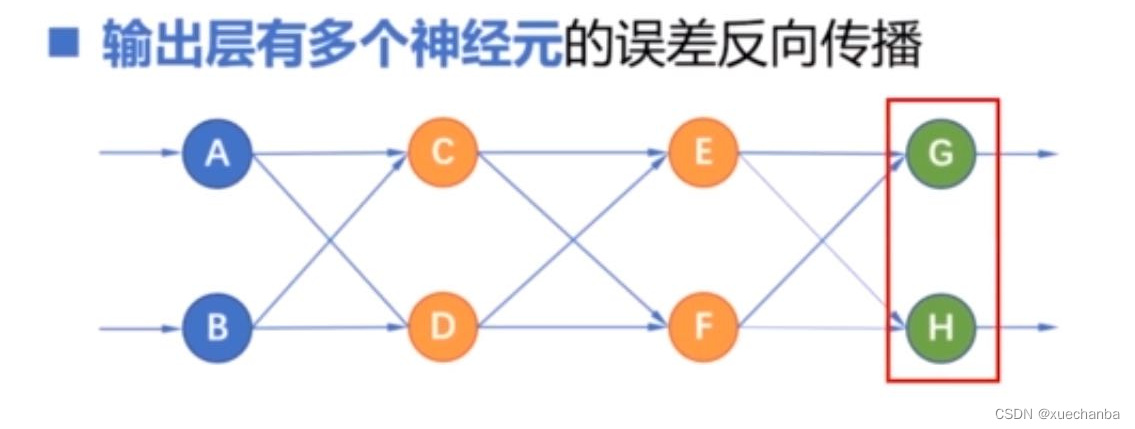那么输出层结点 G 的误差分别传递给节点 E 和结点 F ，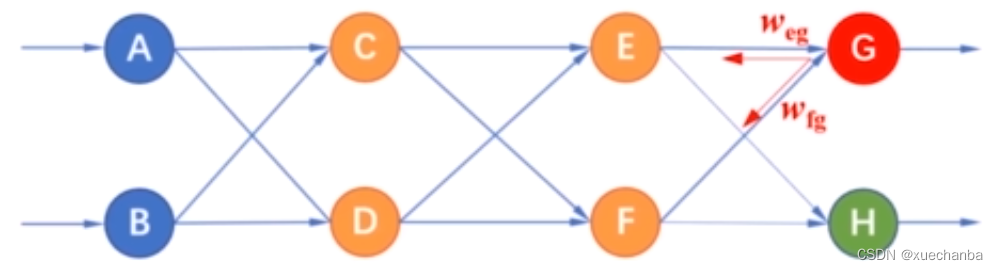而 H 的误差也分别传递给节点 E 和结点 F ，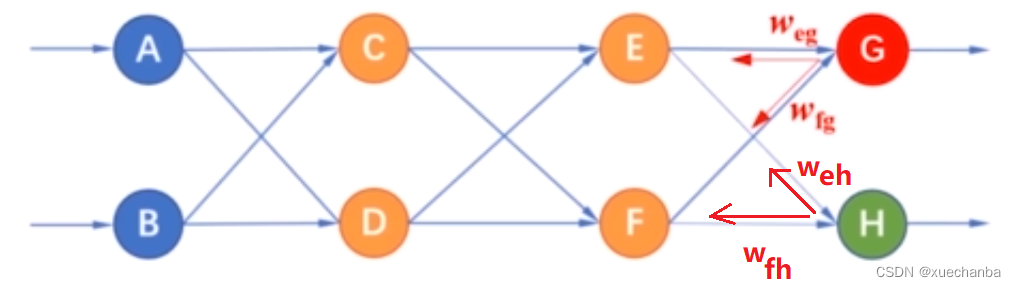下图分别为 E 和 F 接收到的误差，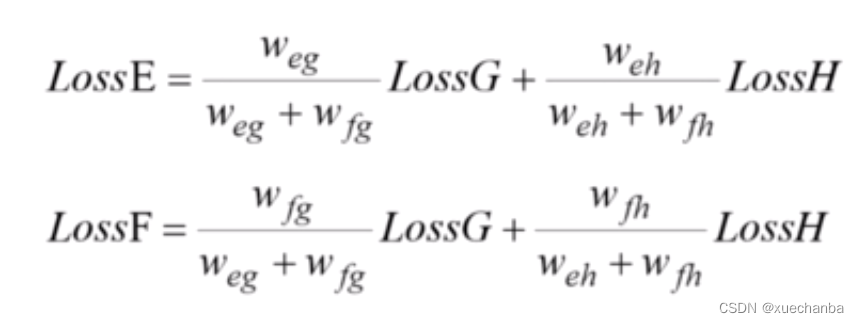继续按照权值比例逐层反向传递误差。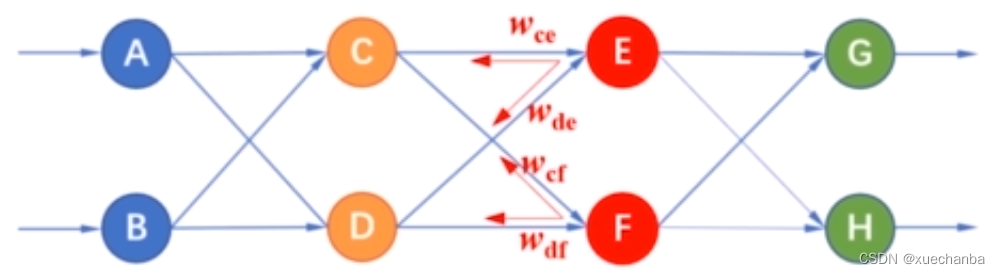可以想象，随着网络层数的加深以及每层中神经元个数的增加，误差反向传播算法得计算也会越来越复杂。

幸运的是，TensorFlow 为我们计算梯度的功能，我们在编程时是不需要这样手动推导公式，并且编写代码实现的。

总结一下，这节课的内容，就是多层神经网络的训练是通过梯度下降法训练模型参数，其中，梯度的计算是通过误差反向传播算法来进行的。训练的过程可以概括为：正向传递信号，反向传递误差。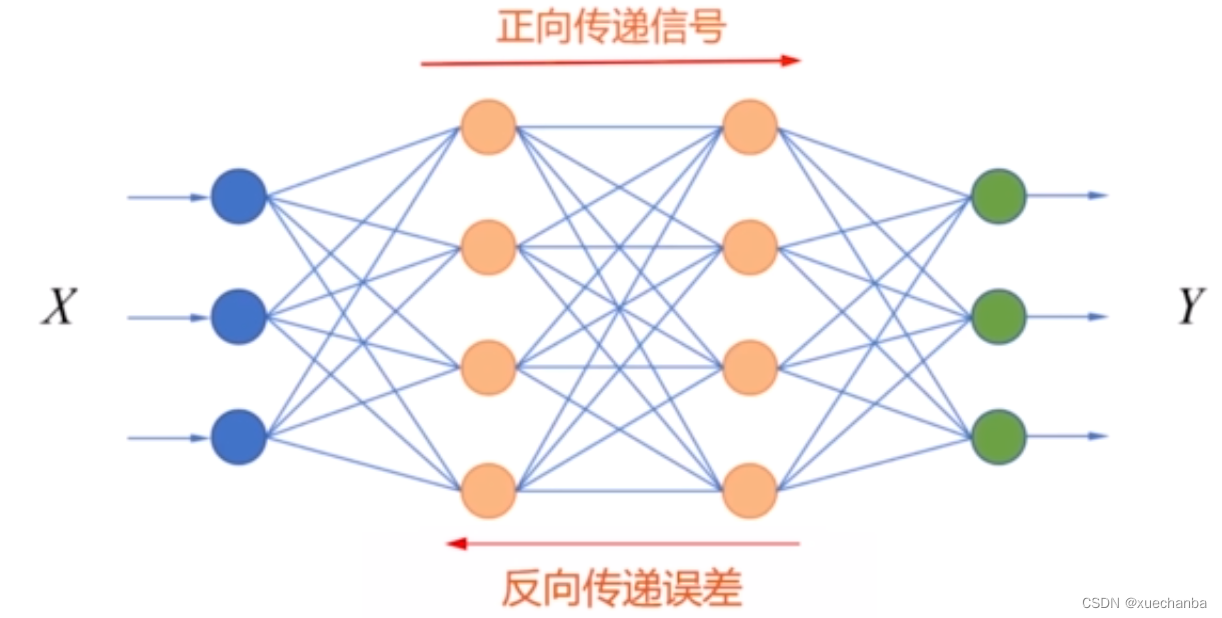首先，在输入层接收输入样本特征 X ，然后在神经网络中逐层传递，直到在输出层得到预测值 Yo 。 接着将神经网络输出的预测值和 Yo 和标签值 Y 相比较，然后计算损失，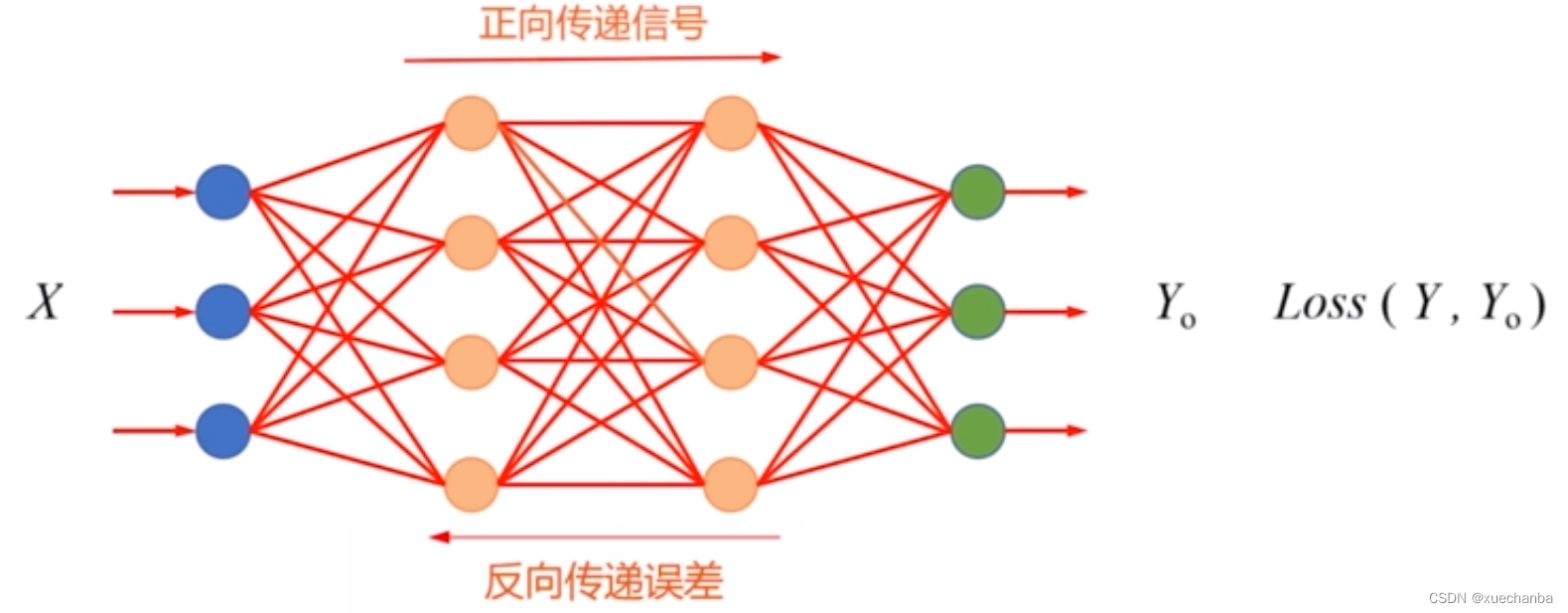如果损失值比较大，就使用梯度下降法调整最后一层神经元的参数，然后反向传播梯度信息，逐层后退，更新模型参数，完成一轮训练。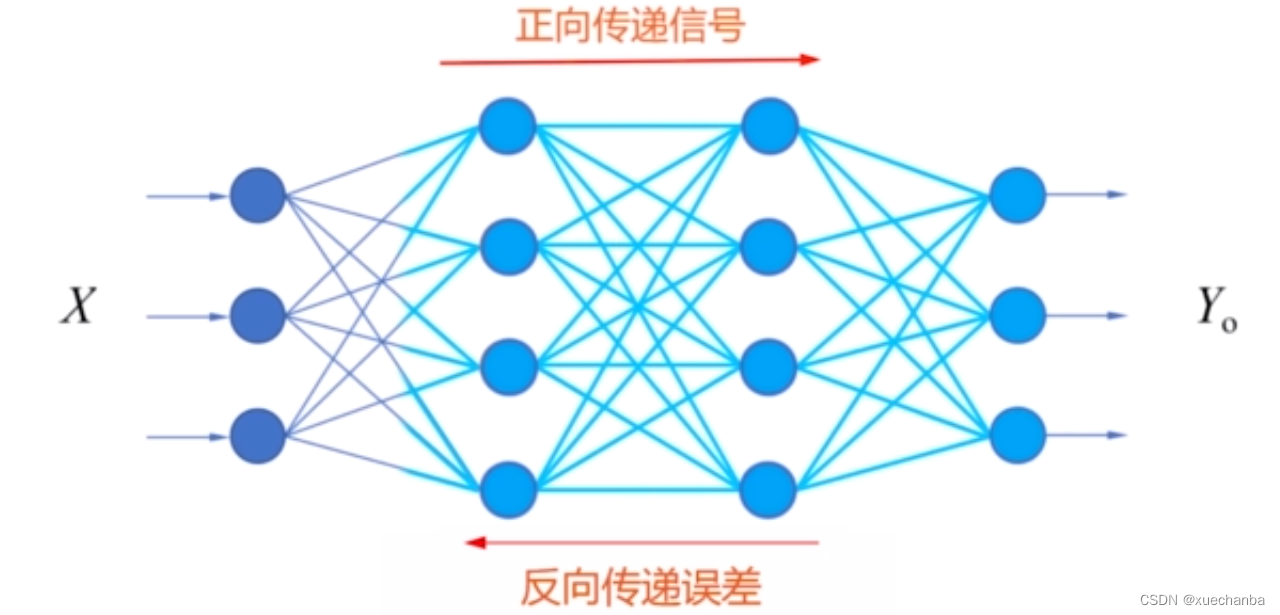参数调整后的网络，再次根据样本特征，正向计算预测值，反向传播误差，调整模型参数，最终通过这样不断地训练，直到网络输出与标签值一致。

这就好像我们学习，每个人都有自己的学习习惯和学习方法，
比如如何测试，如何写作业，如果做笔记等等，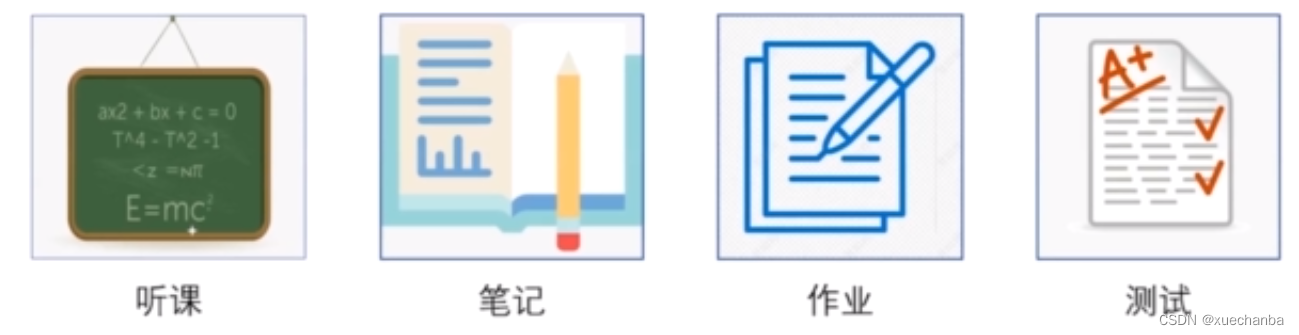我们输入的知识就可以当做是 X ，经过这一系列环节之后，迎来一场考试，这就是知识正向传播的过程，输出就是考试的成绩。

如果没有自动更新的能力，那么这个考试成绩就不会去改变学习习惯，下次再考，还是这个成绩，而如果具备这种误差反向传播的能力，那么就会根据试卷上的错题，分析自己学习方法的不足，然后一层一层倒回去，调整自己的学习方式和学习习惯，完成对学习活动中各个环节的参数的调整，然后再下一轮的学习中就可以表现的更好，得到一个满意的成绩。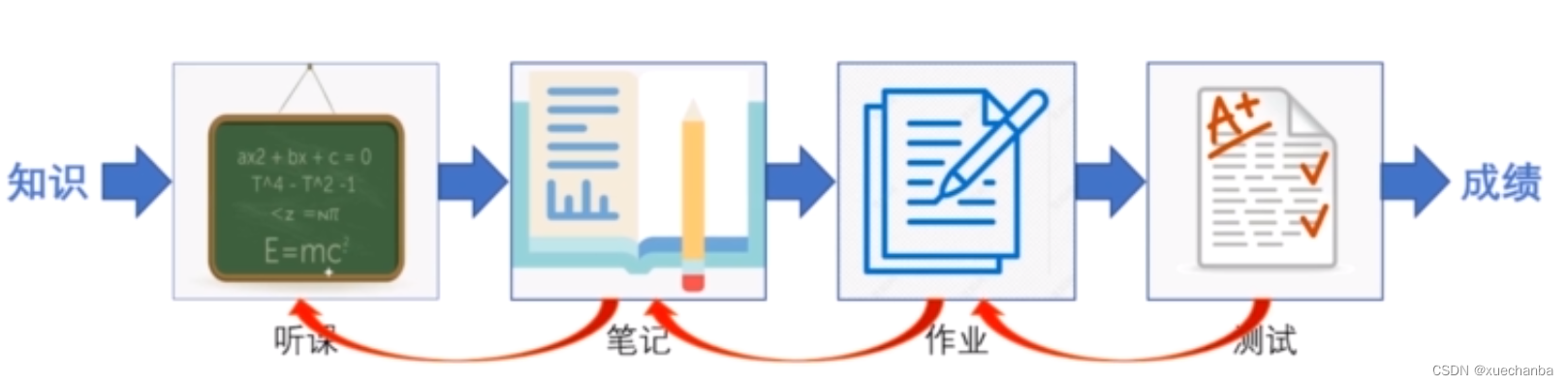如果泛化能力很强，那么使用这套调整后的学习方法再今后学习不同领域的知识时，也能取得一个好成绩。

展开全文算法 神经网络 深度学习
• ## 误差反向传播

千次阅读 2019-06-19 09:00:00
误差反向传播，就是其中最为高效的一种求解导数的算法。   但是，误差反向传播并不是很容易被理解。 作者是数学专业出身，也花了不少功夫来理解这个东西。完了就在思考，明明就是一个复合函数的链式求导，为毛会...

点击上方“大数据与人工智能”，“星标或置顶公众号”

第一时间获取好内容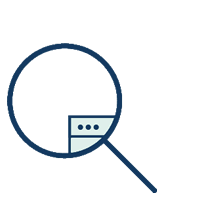作者丨stephenDC

这是作者的第11篇文章

前馈网络是神经网络中最为基础的一种，对网络结构的修改可以延伸出其他的网络类型。前馈网络模型的训练，通常基于模型参数的导数。

而误差反向传播，就是其中最为高效的一种求解导数的算法。

但是，误差反向传播并不是很容易被理解。

作者是数学专业出身，也花了不少功夫来理解这个东西。完了就在思考，明明就是一个复合函数的链式求导，为毛会整的这么难以理解呢？

后来发现，其实是“误差反向传播”这个概念有一定的误导性，如果当初起名叫“导数反向传播”或者“梯度反向传播”，就容易理解多了。

本文就跟大家聊一下这个话题。

前馈网络模型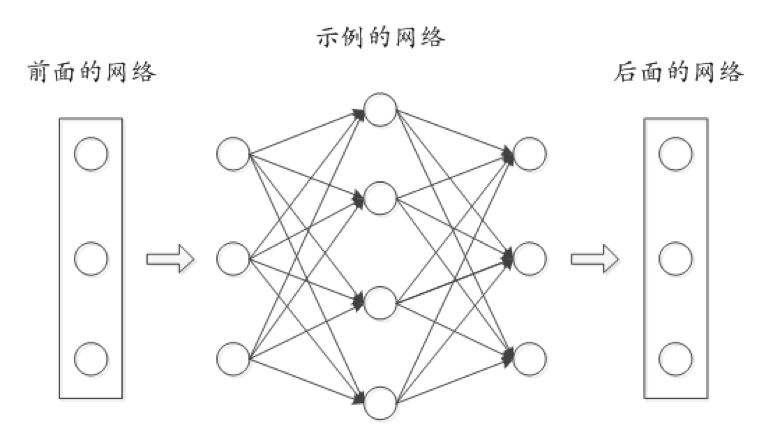图1. 前馈网络结构

前馈网络的结构，如上图所示。

其中包括两类对象，结点和边。结点用来表示一个变量，而边则表示两个结点直接的关系。图中的边我们画成了有向的（带箭头），这是因为从输入到输出，信息是单向的向前流动的，而不能反向流动，所谓的“前馈”，也正是这个意思。每一条边上会附带一个参数，称之为“权重”，其作用我们后面再提。

为了一般性，我们在上图中把网络分成了三个部分，中间部分拿来作为示例，其前后都可能还有若干层的网络结构。

在很多文献里，会把结点称为“神经元”，然后讲“树突”、“轴突”什么的。尽管神经网络模型的提出，确实受到了神经科学的启发，但学习这个模型的时候最好忘掉这些东西，没有什么鸟用。

原因有两点：

1. 作为一个抽象出来的数学模型，应该更加通用，跟生物和神经科学扯在一起，只会限制你的思考。

2. 真正生物意义上的“神经网络”，其结构远比这个复杂，这个模型并不足以描述。

模型的计算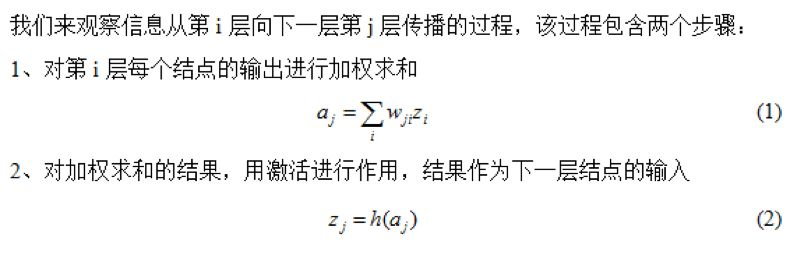很简单对吧？

不过这里要注意的有两点：

1.  对于回归问题，神经网络的输出层（也即最后一层），通常采用线性激活函数，而中间层则采用非线性激活函数。这里为了说明方便，采用了统一的激活函数。

2.  从输入到输出，每一层都先后按照以上方式进行作用，其结果就是输出变成了输入的一个复合函数。信息每向前传播一层，复合函数之上就多加了一次线性加权求和和一次激活函数作用。

模型的训练

对于监督学习来说，计算出损失函数，然后求出损失函数对各个模型参数的导数，即可对模型进行训练。

对神经网络模型而言，参数求导似乎是一个非常艰巨的任务，一是因为模型参数非常多；二是因为复合函数非常复杂。

事实上确实如此，但幸运的是，模型参数的导数之间是有关系的，利用这种关系可以高效地对所有参数进行求导。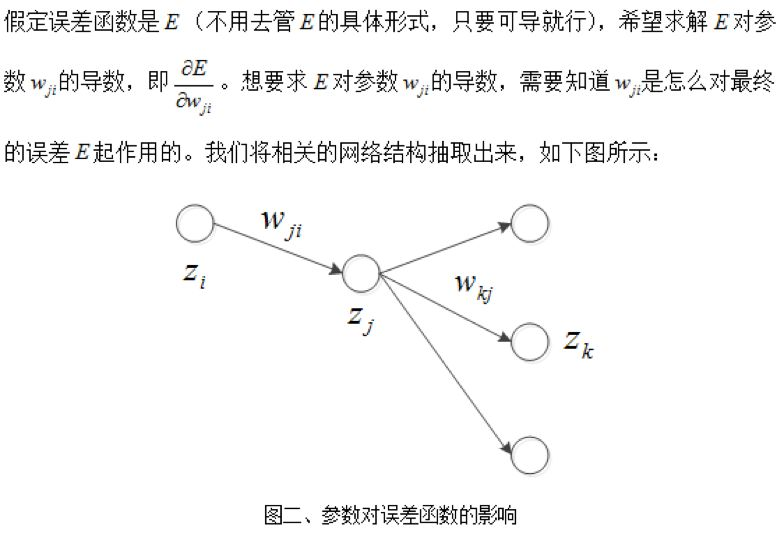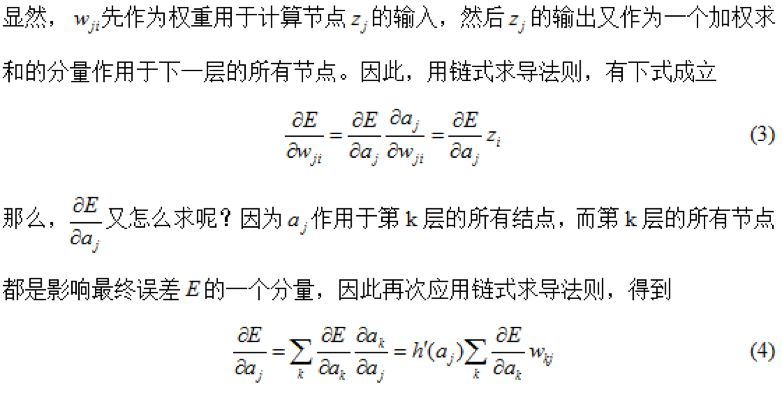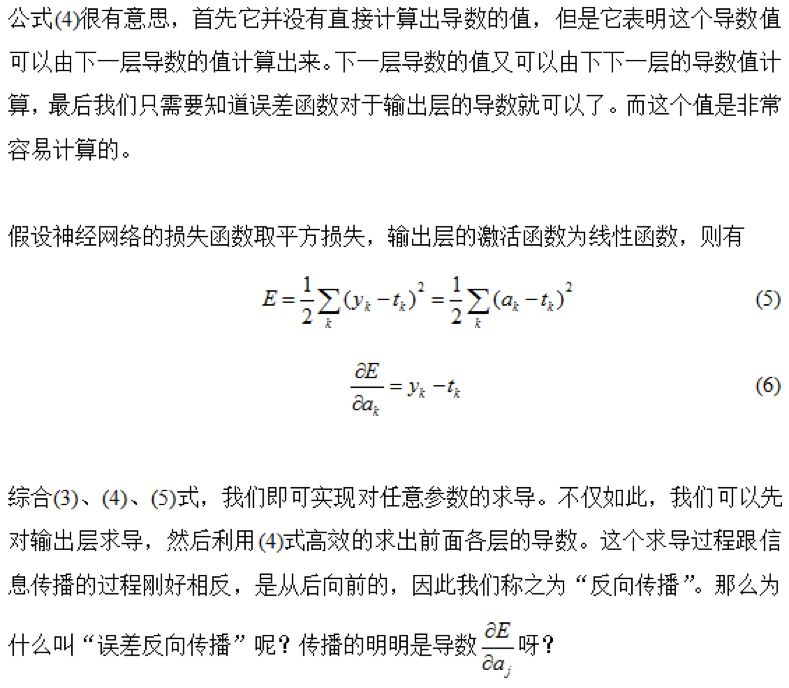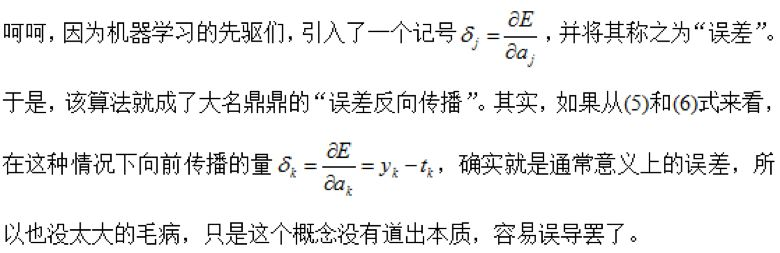小结

这篇文章告诉我们，如果有一天你成了大神，发明了什么算法，一定要慎重地给各种相关概念起名字。

大神们都知道咋回事，但容易误导他人啊！谁特么告诉我，输出为连续值的监督学习问题，怎么就叫Regression了？（摊手...）

-end-展开全文• 目前，深度学习采用的模型主要是神经网络模型，其主要原因是神经网络模型可以使用误差反向传播算法，从而可以比较好地解决贡献度分配问题。只要是超过一层的神经网络都会存在贡献度分配问题，因此可以将超过一层的...

复习一下基础。

#### 一、机器学习模型的处理方法

（1） 数据预处理： 经过数据的预处理，如去除噪声等。比如在文本分类中，去除停用词等。
（2） 特征提取：从原始数据中提取一些有效的特征。比如在图像分类中，提取边缘、尺度不变特征变换（Scale Invariant Feature Transform, SIFT）
（3）特征转换：对特征进行一定的加工，比如降维和升维。降维包括特征抽取（Feature Extraction）和特征选择（Feature Selection）两种途径。常用的特征转换方法有主成分分析、线性判别分析等。
（4）预测： 机器学习的核心部分，学习一个函数并进行预测。
NOTE: 开发一个机器学习系统的主要工作量都消耗在了预处理、特征提取以及特征转换上。

#### 二、深度学习

深度学习是机器学习的一个子问题，其主要目的是从数据中自动学习到有效的特征表示。
经过多层的特征转换，把原始数据变成更高层次、更抽象的表示。这些学习到的表示可以替代人工设计的特征，从而避免“特征工程”。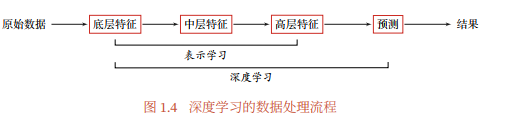深度学习是将原始的数据特征通过多步的特征转换得到的一种特征表示，并进一步输入到预测函数得到最终结果。和“浅层学习”不同，深度学习需要解决的关键问题是贡献度分配问题，即一个系统中不同的组件或其参数对最终系统输出结果的贡献或影响。
目前，深度学习采用的模型主要是神经网络模型，其主要原因是神经网络模型可以使用误差反向传播算法，从而可以比较好地解决贡献度分配问题。只要是超过一层的神经网络都会存在贡献度分配问题，因此可以将超过一层的神经网络都看做深度学习模型。

#### 三、常用的神经网络结构

1、前馈网络（MLP）
前馈网络中各个神经元按接收信息的先后分为不同的组，每一组可以看做一个神经层。每一层中的神经元接收前一层神经元的输出，并输出到下一层神经元。整个网络的信息是朝着一个方向传播，没有反向传播，可以用一个有向无环图表示。（eg. 全连接前馈网络、CNN）。前馈网络可以看作一个函数，通过简单非线性函数的多次复合，实现输入空间到输出空间的复杂映射。这种网络结构简单，易于实现。
在前馈神经网络中，各神经元分别属于不同的层。每一层的神经元可以接收前一层神经元的信号，并产生信号输出到下一层。第0层称为输入层，最后一层称为输出层，其他中间层称为隐藏层。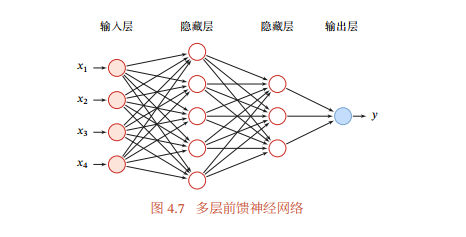2、记忆网络
也称为反馈网络，网络中的神经元不但可以接受其他神经元的信息，也可以接收自己的历史信息。和前馈网络相比，极意网络中的神经元具有记忆功能，在不同的时刻具有不同的状态。记忆网络中的信息传播可以是单向或双向传递，因此可用一个有向循环图或无向图来表示。（RNN、Hopfield网络、玻尔兹曼机、受限玻尔兹曼机等）。
3、图网络
图网络是定义在图结构数据上的神经网络，图中每个节点都由一个或一组神经元构成。节点之间的连接可以是有向的，也可以是无向的。每个节点可以收到来自相邻节点或者自身的信息。（知识图谱、社交网络、分子网络）。一些实现方式：图卷积网络、图注意力网络、消息传递神经网络等。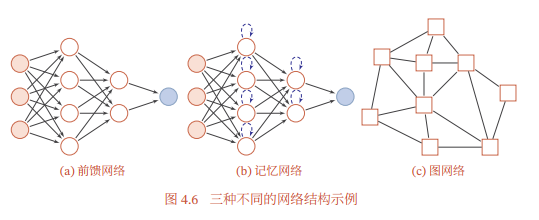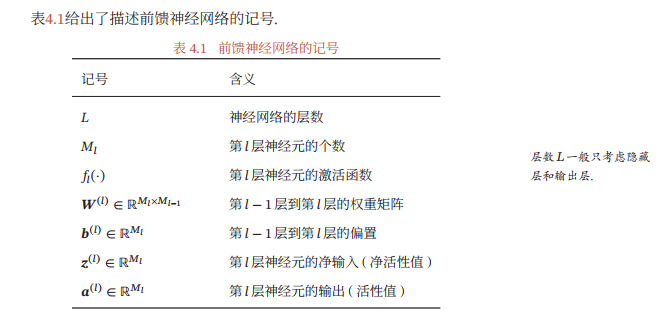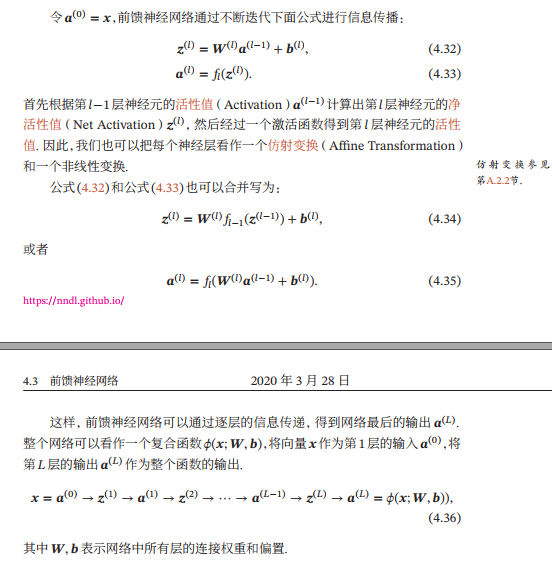#### 四、参数学习

如果采用交叉熵损失函数，对于样本（x,y)，其损失函数为：
L ( y , y ^ ) = − y T l o g y ^ \mathcal{L}(y, \hat{y})=-y^Tlog\hat{y}
其中 y ∈ { 0 , 1 } C y\in \{0,1\}^C 为标签y对应的one-hot向量表示。
给定训练集为 D = { ( x ( n ) , y ( n ) ) } n = 1 N \mathcal{D}=\{(x^{(n)},y^{(n)})\}_{n=1}^N ,，将每个样本 x ( n ) x^{(n)} 输入给前馈神经网络，得到网络输出为 y ^ n \hat{y}^{n} ，其在数据集 D \mathcal{D} 上的结构化风险函数为：
R ( W , b ) = 1 N ∑ n = 1 N L ( y ( n ) , y ^ ( n ) ) + 1 2 λ ∣ ∣ W ∣ ∣ F 2 \mathcal{R}(W,b)=\frac{1}{N}\sum_{n=1}^{N}\mathcal{L}(y^{(n)},\hat{y}^{(n)})+\frac{1}{2}\lambda ||W||_F^2
其中W和b分别表示网络中所有的权重矩阵和偏置向量；||W||_F^2是正则化项，用来防止过拟合； λ > 0 \lambda > 0 为超参数， λ \lambda 越大，W越接近于0,。这里的 ∣ ∣ W ∣ ∣ F 2 ||W||_F^2 一般使用Frobenius范数。
∣ ∣ W ∣ ∣ F 2 = ∑ l = 1 L ∑ i = 1 M l ∑ j = 1 M i − 1 ( w i j ( l ) ) 2 ||W||_F^2=\sum_{l=1}^{L}\sum_{i=1}^{M_l}\sum_{j=1}^{M_{i-1}}(w_{ij}^{(l)})^2
有了学习准则和训练样本，网络参数可以用过梯度下降法来进行学习。在梯度下降方法的每次迭代中，第 l l 层的参数 W ( l ) W^{(l)} b ( l ) b^{(l)} 参数更新方式为：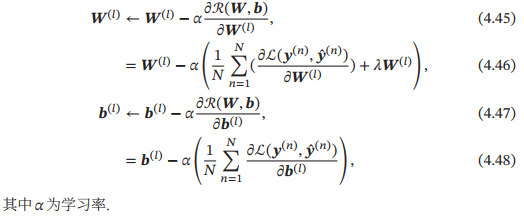如果通过链式法则逐一对每个参数进行求偏导数比较低效。在神经网络的训练中经常使用反向传播算法来高效的计算梯度。

#### 五、反向传播算法

假设采用随机梯度下降进行神经网络参数学习，给定一个样本（x,y），将其输入到神经网络模型中，得到网络输出为 y ^ \hat{y} 。假设损失函数为 y , y ^ \mathcal{y,\hat{y}} ，要进行参数学习就需要计算损失函数关于每个参数的导数。
不失一般性，对第l层中的参数 W ( l ) W^{(l)} b ( l ) b^{(l)} 计算偏导数。因为 ∂ L ( y , y ^ ) ∂ W ( l ) \frac{\partial \mathcal{L}(y,\hat{y})}{\partial W^{(l)}} 的计算涉及向量对矩阵的微分，十分繁琐，因此我们先计算 L ( y , y ^ ) \mathcal{L}{(y,\hat{y})} 关于参数矩阵中每个元素的偏导数 ∂ L ( y , y ^ ) ∂ w i j ( l ) \frac{\partial \mathcal{L}(y,\hat{y})}{\partial w_{ij}^{(l)}} 。根据链式法则，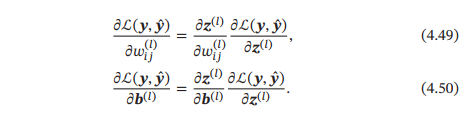上式中第二项都为目标函数关于第 l l 层的神经元 z ( l ) z^{(l)} 的偏导数，称为误差项，可以一次计算得到。这样我们只需要计算三个偏导数，分别为 ∂ z ( l ) ∂ w i j ( l ) \frac{\partial z^{(l)}}{\partial{w_{ij}}^{(l)}} ∂ z ( l ) ∂ b ( l ) \frac{\partial z^{(l)}}{\partial{b}^{(l)}} ∂ L ( y , y ^ ) ∂ z ( l ) \frac{\partial \mathcal{L}(y,\hat{y})}{\partial z^{(l)}}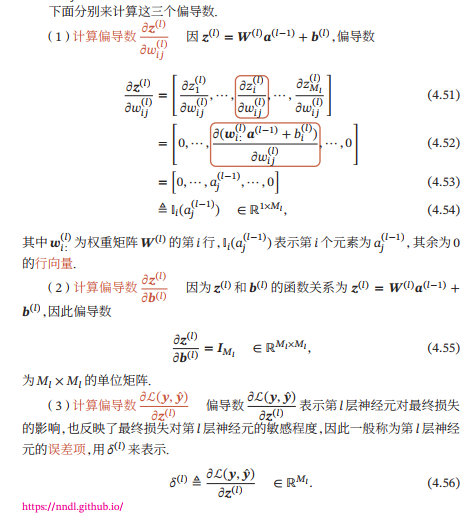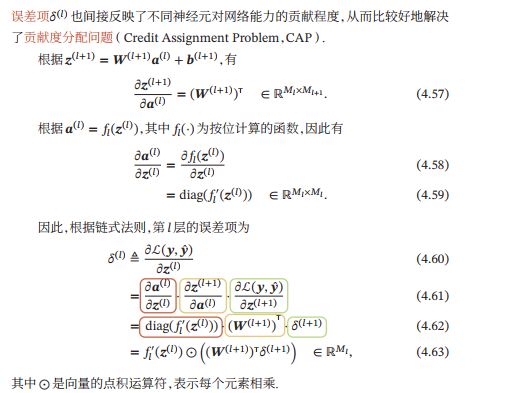从上式可以看出，第l层的误差项可以通过第 l + 1 l+1 层的误差项计算得到，这就是误差的反向传播（BackPropagation,BP）。
反向传播算法的含义是：第l层的一个神经元的误差项（敏感性）是所有与该神经元相连的第l+1层的神经元的误差项的权重和。然后，再乘上该神经元激活函数的梯度。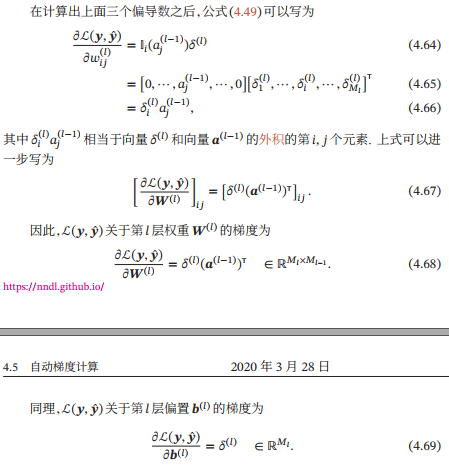在计算出每一层的误差项之后，就可以得到每一层参数的梯度。因此，使用误差反向传播算法的前馈神经网络训练过程可以分为以下三步：
（1）前馈计算每一层的净输入 z ( l ) z^{(l)} 和激活值 a ( l ) a^{(l)} ，直到最后一层；
（2）反向传播计算每一层的误差项 δ ( l ) \delta^{(l)}
(3)计算每一层参数的偏导数，并更新参数。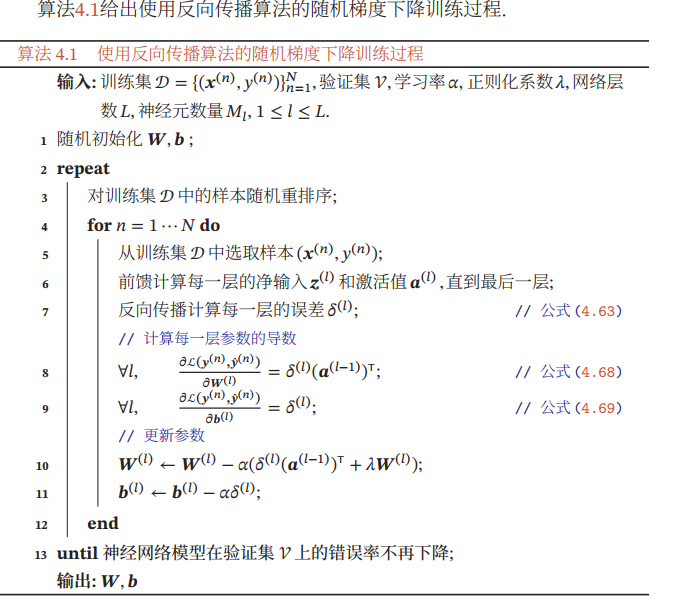#### 六、自动梯度计算

分为三类： 数值微分、符号微分和自动微分
（1）数值微分： 使用数值方法来计算函数 f ( x ) f(x) 的导数。实用性较差，计算复杂度较高。
（2）符号微分：基于符号计算的自动求导方法。符号计算也叫代数计算，是指用计算机来处理带有变量的数学表达式。
（3） 自动微分： 基本原理式所有的数值计算可以分解为一些基本操作，包含+、-，*，/和一些初等函数，exp,log,sin,cos等，然后利用链式法则来自动计算一个复合函数的梯度。

• 静态计算图和动态计算图
静态计算图是在编译时构建计算图，计算图构建好之后在程序运行时不能改变，而动态计算图是在程序运行时动态构建。两种构建方式各有优缺点。静态计算图在构建时可以进行优化，并行能力强，但灵活性比较差。动态计算图则不容易进行优化，当不同的输入的网络结构不一致时，难以并行计算，但是灵活性比较高。

#### 参考文献

 神经网络与深度学习. 邱锡鹏.

展开全文算法 深度学习 神经网络
• 深度学习：基于python第5章 误差反向传播法5.1 计算图5.1.1　用计算图求解5.1.2　局部计算5.1.3　为何用计算图解题5.2 链式法则5.2.1 计算图的反向传播5.2.2　什么是链式法则5.2.3　链式法则和计算图*5.3 反向传播...python 开发语言
• 目录神经网络与误差反向传播算法1 神经网络1.1 神经网络1.2 神经网络的作用1.3 神经元模型1.4 人工神经网络1.5 前馈神经网络2 Delta学习规则3 梯度3.1 梯度下降3.2 梯度下降示意3.3 输出层权重改变量3.4 隐藏层权重...神经网络 算法 深度学习
• 1. 计算图 计算图将计算过程用图形...反向传播（backward propagation）：是从右向左进行计算，传递的是局部导数。 局部计算：计算图的特征是可以通过传递“局部计算”获得最终结果。“局部”这个词的意思是“与自...深度学习 神经网络 人工智能 算法
• ## 详解 误差反向传播算法推导

万次阅读 多人点赞 2018-09-14 03:58:31
误差反向传播算法误差 反向传播算法（back propagation，简称BP模型）是1986年由Rumelhart和McClelland为首的科学家提出的概念，是一种按照误差逆向传播算法训练的多层前馈神经网络，是目前应用最广泛的神经网络...BP网络
• 五、误差反向传播法 这次用到的代码上传到csdn了，0积分下载误差反向传播代码 其实本文也都贴出来了，只是需要改一下import的路径 还有一些数学公式函数数学公式 若理解困难参考上一章节神经网络的学习-搭建神经网络...深度学习 人工智能 机器学习
• 输入学习样本，使用反向传播算法对网络的权值和偏差进行反复的调整训练，使输出的向量与期望向量尽可能地接近，当网络输出层的误差平方和小于指定的误差时训练完成，保存网络的权值和偏差。
• 误差反向传播是指，对于每次求出的Loss（损失），通过链式求导法则和梯度下降法，更新所有要学习的参数。 例如，对于线性模型： y^=x∗ω+b\hat{y}=x*\omega+by^​=x∗ω+b 其可训练的参数就是ω\omegaω与bbb。 ...
• 1、计算图：将计算过程用数据结构图表示，通过多个节点和边表示，节点用O表示，O中是计算内容，将计算的中间结果写在箭头上方，表示各个节点的计算结果从左向右传递...②可以通过反向传播高效计算导数，反向传播使用与神经网络 深度学习 python
• 反向传播算法又称误差反向传播、BP算法( Backpropagation,缩写为BP）。 BP算法的学习过程由正向传播过程和反向传播过程组成。 在正向传播过程中，输入信息通过输入层经隐含层，逐层处理并传向输出层。如果在输出层得...
• 鱼书中要求在方块中填入数字，完成反向传播练习题的计算过程。人工智能 深度学习 神经网络
• 在机器学习算法中，误差反向传播和梯度下降是模型收敛的关键技术，作者将以单隐层模型为例说明误差反向传播和梯度下降的工作原理。 各种算法的单隐层模型结构大同小异 正向传播 假设：主要模型参数有3个，输入...机器学习 梯度下降 算法原理
• 实际上，反向传播算法就是追踪权重(和偏置)的这种微小的变化是如何影响到损失函数的技术。 反向传播算法的特点是效率高。反向传播可以同时计算所有的偏导数，仅仅使用一次前向传播，加上一次后向传播。假设对一个...机器学习 人工智能 深度学习 神经网络 AI
• 误差反向传播（Error Back Propagation, BP）算法 1、BP算法的基本思想是：学习过程由信号的正向传播与误差的反向传播两个过程组成。 1）正向传播：输入样本－>输入层－>隐藏层（处理）－>输出层 举一个...
• 随机梯度下降和误差反向传播是BP神经网络和卷积神经网络的核心。 随机梯度下降，通俗来说，就是对于给定的目标损失函数，找到一个可以迭代的式子，通过不停地迭代，能够找到函数的极值（可能会陷入局部最优解） ...神经网络 深度学习 机器学习
• 2.1概述 ​ 反向传播算法的全称是误差反向传播算法，其本质通过总的误差函数对每一个权重和偏置求偏导数，再通过偏导数更新权值和偏置，多次重复此步骤以找到最佳的偏置和权重值。（其原理是偏导数反应了某个函数值...神经网络 算法 深度学习
• 深度学习入门-误差反向传播法 摘要 通过使用计算图，可以直观地把握计算过程。 计算图的节点是由局部计算构成的。局部计算构成全局计算。 计算图的正向传播进行一般的计算。通过计算图的反向传播，可以计算...
• 二是整理前馈神经网络中正向传播、误差反向传播和梯度下降的原理；三是梯度消失和梯度爆炸问题的原因及解决思路。 一、神经网络结构 目前比较常用的神经网络结构有如下三种： 1、前馈神经网络 前馈神经网络中，...
• 文章目录一、BP算法理解二、BP算法数据模型三、误差反向传播代码实现 一、BP算法理解 ① BP算法全称 (Error) Back Propagation 算法，中文名曰：误差反向传播算法。 ②该算法是干嘛的？ 计算梯度。更简单点，求(偏)...算法 神经网络 深度学习
• 误差反向传播法 步骤 准备好学习数据。 进行权重和偏置的初始设置，设置学习率。 计算神经单元的输出值及平方误差C。 根据误差反向传播法，计算各层的神经单元误差。 根据神经单元误差计算平方误差C的偏导数。 计算...深度学习 神经网络
• 一、误差反向传播神经网络的提出 采用非线性连续变换函数，使隐含层神经元具有学习能力。 二、误差反向传播神经网络结构 互连结构 BP神经网络是一种具有三层或三层以上结构的无反馈的层内无互联的前向网络，各层...神经网络
•python 深度学习 神经网络 机器学习 算法
• 误差反向传播网络（Error Back Propagtion，），简称为BP神经网络，是一种多层神经网络，与之相关的另一个概念是多层感知器（Multi-Layer Perceptron，MLP）。多层感知器除了输入层和输出层以外，还具有若干个隐含层...人工智能 神经网络
• 1.误差反向传播算法是干什么的？ 通过数值微分计算了神经网络的权重参数的梯度，数值微分虽然简单，也容易实现，但缺点是计算上比较费时间。本章我们将学习一个能够高效计算权重参数的梯度的方法——误差反向传播法...
• 关于反向传播，我非常推荐下面的一个在线书籍，其给出了非常详实、生动且易于理解的反向传播算法以及相关公式的推导，链接为：Neural Networks and Deep Learning。本文将对其讲解给以归纳和总结，其中不乏添加一些...神经网络 深度学习 机器学习...